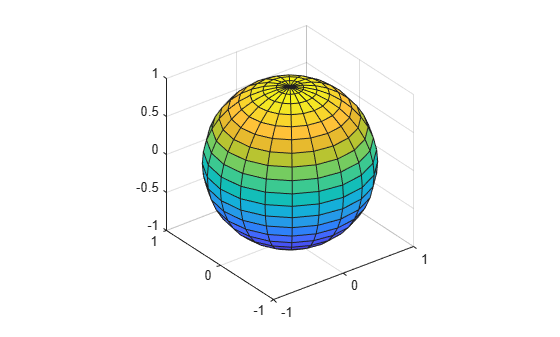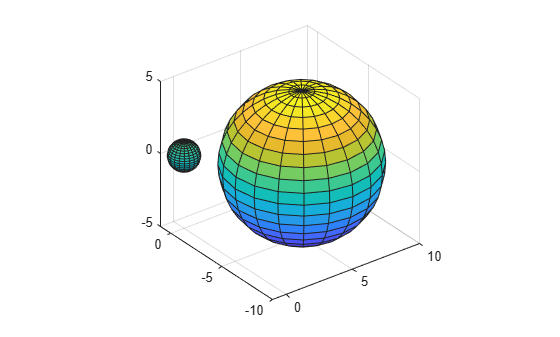# sphere

## Syntax

``[X,Y,Z] = sphere``
``[X,Y,Z] = sphere(n)``
``sphere(___)``
``sphere(ax,___)``

## Description

example

````[X,Y,Z] = sphere` returns the x-, y-, and z- coordinates of a sphere without drawing it. The returned sphere has a radius equal to `1` and consists of 20-by-20 faces. The function returns the x-, y-, and z- coordinates as three `21`-by-`21` matrices.To draw the sphere using the returned coordinates, use the `surf` or `mesh` functions.```

example

````[X,Y,Z] = sphere(n)` returns the x-, y-, and z- coordinates of a sphere with a radius equal to `1` and `n`-by-`n` faces. The function returns the x-, y-, and z- coordinates as three `(n+1)`-by-`(n+1)` matrices.```

example

````sphere(___)` plots the sphere without returning the coordinates. Use this syntax with any of the input arguments in previous syntaxes.```

example

````sphere(ax,___)` plots into the axes specified by `ax` instead of the current axes. Specify the axes as the first input argument.```

## Examples

collapse all

Create and plot a sphere with a radius equal to 1. Use `axis equal` to use equal data units along each coordinate direction.

```sphere axis equal```Specify the radius and location of a sphere by modifying the returned `X`, `Y`, and `Z` coordinates.

Define `X`, `Y`, and `Z` as coordinates of a unit sphere.

`[X,Y,Z] = sphere;`

Plot the unit sphere centered at the origin.

```surf(X,Y,Z) axis equal```Define `X2`, `Y2`, and `Z2` as coordinates of a sphere with a radius of 5 by multiplying the coordinates of the unit sphere. Plot the second sphere, centering it at `(5,-5,0)`.

```hold on r = 5; X2 = X * r; Y2 = Y * r; Z2 = Z * r; surf(X2+5,Y2-5,Z2)```Call the `tiledlayout` function to create a 2-by-2 tiled chart layout. Call the `nexttile` function to create the axes. Then, use the `sphere` function to plot three spheres with different numbers of faces into different tiles of the chart by specifying the axes.

```tiledlayout(2,2); ax1 = nexttile; sphere(ax1); axis equal title('20-by-20 faces (Default)') ax2 = nexttile; sphere(ax2,50) axis equal title('50-by-50 faces') ax3 = nexttile; sphere(ax3,100) axis equal title('100-by-100 faces')```## Input Arguments

collapse all

Number of faces, specified as a positive integer.

Target axes, specified as an `Axes` object. If you do not specify the axes, then `sphere` plots into the current axes.

## Version History

Introduced before R2006a